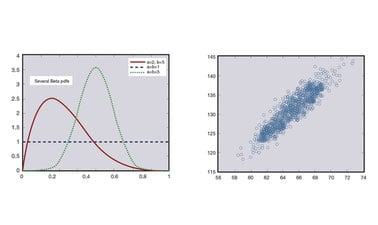Ir al contenido principal

# Probability and Statistics II: Random Variables – Great Expectations to Bell Curves

This course discusses properties and applications of random variables. For instance, how many customers are likely to arrive in the next hour? What’s the probability that a lightbulb will last more than a year?

When you’re done with this course, you’ll have enough firepower to undertake a wide variety of modeling and analysis problems; and you’ll be well-prepared for the upcoming Statistics courses.### Elige tu sesión:

Comienza el 3 dic
Termina el 24 dic
Comienza el 10 ene 2022
Comienza el 16 may 2022
6–12 horas por semana
A tu ritmo
Avanza a tu ritmo
Gratis
Verificación opcional disponible

# Sobre este curso

Omitir Sobre este curso

This course discusses properties and applications of random variables. When you’re done, you’ll have enough firepower to undertake a wide variety of modeling and analysis problems; and you’ll be well-prepared for the upcoming Statistics courses.

We’ll begin by introducing the concepts of discrete and continuous random variables. For instance, how many customers are likely to arrive in the next hour (discrete)? What’s the probability that a lightbulb will last more than a year (continuous)?

We’ll learn about various properties of random variables such as the expected value, variance, and moment generating function. This will lead us to a discussion of functions of random variables. Such functions have many uses, including some wonderful applications in computer simulations.

If you enjoy random variables, then you’ll really love joint (two-dimensional) random variables. We’ll provide methodology to extract marginal (one-dimensional) and conditional information from these big boys. This work will enable us to
study the important concepts of independence and correlation.

Along the way, we’ll start working with the R statistical package to do some of our calculations and analysis.

### De un vistazo

• Institución: GTx
• Tema: Análisis de datos
• Nivel: Intermediate
• Prerrequisitos:

Learners will be expected to come in knowing a bit of set theory and basic calculus, as well as the material from the first course in this series (A Gentle Introduction to Probability). The prerequisite material is all available for you to access; and in any event, we will make the current course as self-contained as possible. In addition, this course will involve a bit of computer programming, so it would be nice to have at least a little experience in something like Excel.

# Lo que aprenderás

Omitir Lo que aprenderás

Upon completion of this course, learners will be able to:

• Identify discrete and continuous random variables
• Describe the properties of random variables, including the expected value, variance, and moment generating function.
• Understand functions of random variables, and how they can be used in computer simulation applications.
• Recognize joint (two-dimensional) random variables and how to extract marginal (onedimensional) and conditional information from them
• Implement the concepts of independence and correlation
• Work with and implement the R statistical package

# Plan de estudios

Omitir Plan de estudios

“FCPS” refers to the free text, A First Course in Probability and Statistics: free access is provided via a PDF file or as a book

Module 1: Univariate Random Variables

• Lesson 1: Introduction (FCPS §2.1)
• Lesson 2: Discrete Random Variables (FCPS §2.2)
• Lesson 3: Continuous Random Variables (FCPS §2.3)
• Lesson 4: Cumulative Distribution Functions (FCPS §2.4)
• Lesson 5: Great Expectations (FCPS §2.5.1)
• Lesson 6: LOTUS, Moments, and Varience (FCPS §2.5.2)
• Lesson 7 [OPTIONAL]: Approximations to E[h(X)] and Var(h(X)) (FCPS §2.5.3)
• Lesson 8: Moment Generating Functions (FCPS §2.6)
• Lesson 9: Some Probability Inequalities (FCPS §2.7)
• Lesson 10: Functions of a Random Variable (FCPS §2.8.1)
• Lesson 11: Inverse Transform Theorem (FCPS §2.8.2)
• Lesson 12 [OPTIONAL]: Honors Bonus Results (FCPS §2.8.3)

Module 2: Bivariate Random Variables

• Lesson 1: Introduction (FCPS §§3.1.1−3.1.3)
• Lesson 2: Marginal Distributions (FCPS §3.1.4)
• Lesson 3: Conditional Distributions (FCPS §3.2)
• Lesson 4: Independent Random Variables (FCPS §3.3.1)
• Lesson 5: Consequences of Independence (FCPS §3.3.2)
• Lesson 6: Random Samples (FCPS §3.3.3)
• Lesson 7: Conditional Expectation (FCPS §3.4.1)
• Lesson 8: Double Expectation (FCPS §3.4.2)
• Lesson 9 [OPTIONAL]: First-Step Analysis (FCPS §3.4.3)
• Lesson 10 [OPTIONAL]: Random Sums of Random Variables (FCPS §3.4.3)
• Lesson 11 [OPTIONAL]: Standard Conditioning Argument (FCPS §3.4.3)
• Lesson 12: Covariance and Correlation (FCPS §3.5.1)
• Lesson 13: Correlation and Causation (FCPS §3.5.2)
• Lesson 14: A Couple of Worked Correlation Examples (FCPS §3.5.3)
• Lesson 15: Some Useful Covariance / Correlation Theorems (FCPS §3.5.4)
• Lesson 16: Moment Generating Functions, Revisited (FCPS §3.6)
• Lesson 17 [OPTIONAL]: Honors Bivariate Functions of Random Variables (FCPS §3.7)

# Preguntas frecuentes

Omitir Preguntas frecuentes

*What if I don’t remember much basic probability?

Answer: You can take the companion course, “A Gentle Introduction to Probability”, which will get you up to speed lickety split!

*Will there be much programming?

Answer: A little, but you’ll be able to handle that in Excel or Matlab or whatever your favorite programming application is. We also go over a couple of R demos, but those will be self-contained.

## ¿Te interesa este curso para tu negocio o equipo?

Capacita a tus empleados en los temas más solicitados con edX para Negocios.